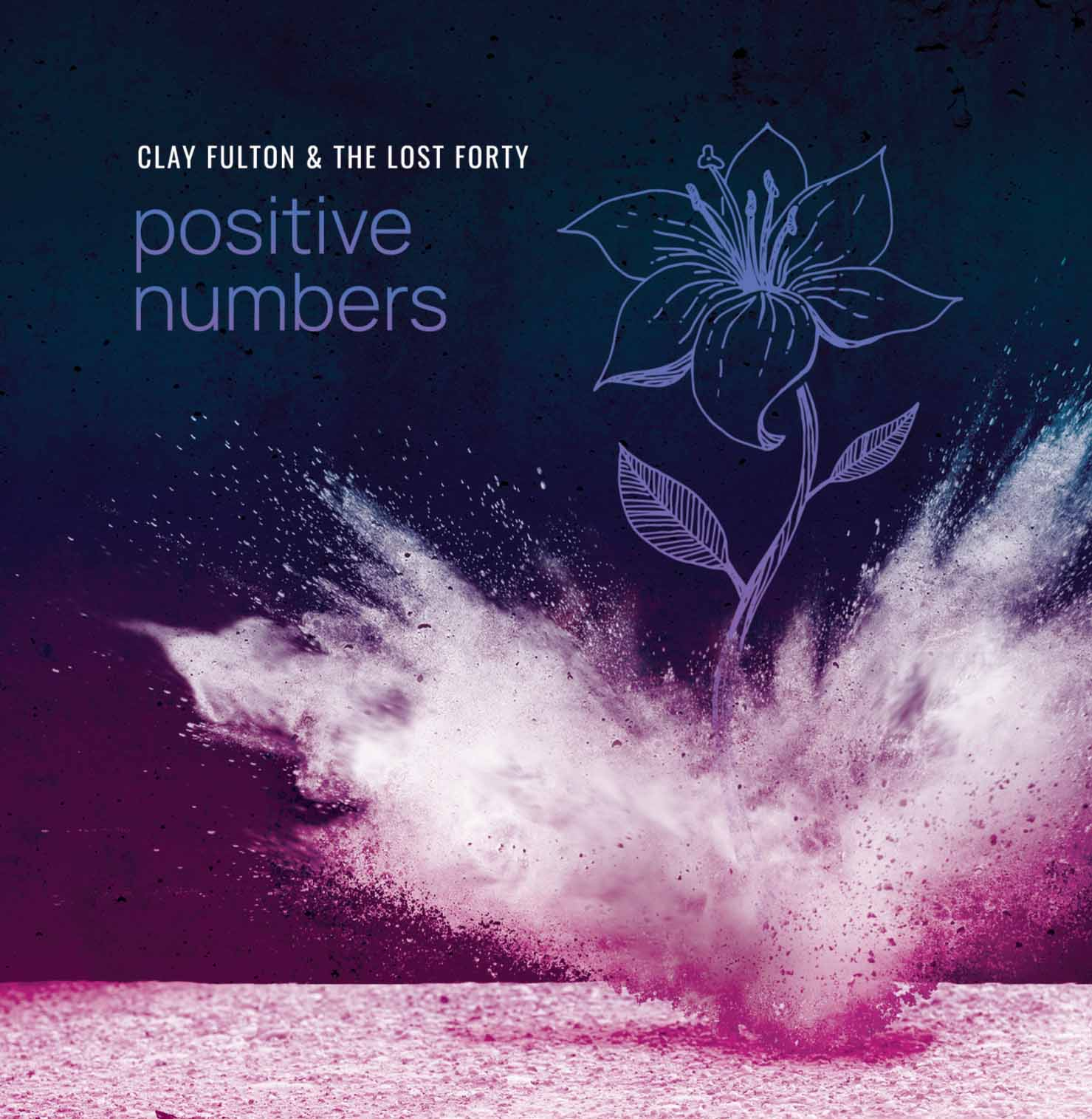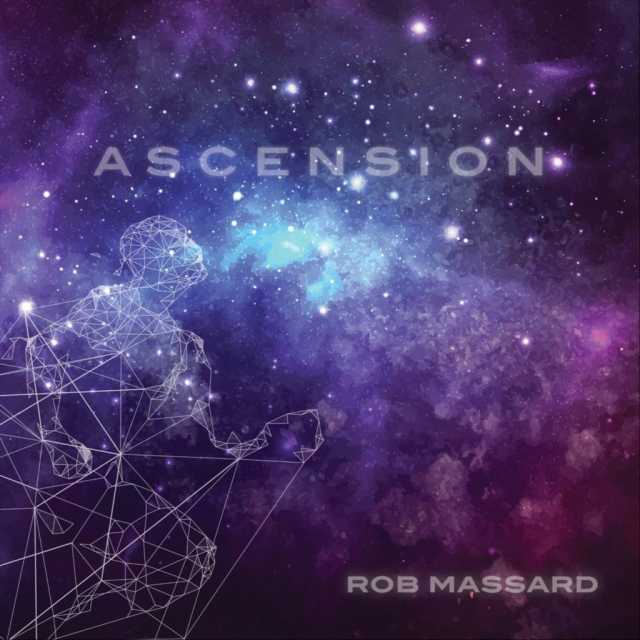Art, History, Music, Politics, and Spirituality For The Modern Alchemist – circulation in over 129 countries

# The Sign of Shamhat Year 18,002: Begins May 14th 2014

There are eight months in the Calendar of Mu, sometimes referred to as the Nyarzirian Calendar. Each month is consists of forty-five days, and represents a system of the eight hexagrams moving through the five elements. The Ninzuwu Priest/Priestess invokes the mudra/mantra formulae associated with each hexagram that rules a particular day after invoking the Armor of Amaterasu Ohkami. The chart below illustrates the daily Vasuh letters invoked and the ruling “deity” for the said hexagram:
1. May 14th 2014 = Aozu = 17th Hexagram = Phe-Hmu-Tuu
2. May 15th 2014 = Asakhira = 28th Hexagram = Aum-Nzu-Bnhu
3. May 16th 2014 = Zwa = 31st Hexagram = Aum-Aum-Hmu
4. May 17th 2014 = Chuki = 43rd Hexagram = Zhee-Zhee-Phe
5. May 18th 2014 = Lalui = 45th Hexagram = Nzu-Hmu-Lewhu-Tuu-Nzu
6. May 19th 2014 = Nhu = 47th Hexagram = Shki-Zhee-Bnhu
7. May 20th 2014 = Butz = 49th Hexagram = Hmu-Shki-Aum
8. May 21st 2014 = Shamhat = 58th Hexagram = Bnhu-Aum
9. May 22nd 2014 =Entering Earth Element
10. May 23rd 2014 = Aozu = 17th Hexagram = Phe-Hmu-Tuu
11. May 24th 2014 = Asakhira = 28th Hexagram = Aum-Nzu-Bnhu
12. May 25th 2014 = Zwa = 31st Hexagram = Aum-Aum-Hmu
13. May 26th 2014 = Chuki = 43rd Hexagram = Zhee-Zhee-Phe
14. May 27th 2014 = Lalui = 45th Hexagram = Nzu-Hmu-Lewhu-Tuu-Nzu
15. May 28th 2014 = Nhu = 47th Hexagram = Shki-Zhee-Bnhu
16. May 29th 2014 = Butz = 49th Hexagram = Hmu-Shki-Aum
17. May 30th 2014 = Shamhat = 58th Hexagram = Bnhu-Aum
18. May 31st 2014 =Entering Water Element
19. June 1st 2014 = Aozu = 17th Hexagram = Phe-Hmu-Tuu
20. June 2nd 2014 = Asakhira = 28th Hexagram = Aum-Nzu-Bnhu
21. June 3rd 2014 = Zwa = 31st Hexagram = Aum-Aum-Hmu
22. June 4th 2014 = Chuki = 43rd Hexagram = Zhee-Zhee-Phe
23. June 5th 2014 = Lalui = 45th Hexagram = Nzu-Hmu-Lewhu-Tuu-Nzu
24. June 6th 2014 = Nhu = 47th Hexagram = Shki-Zhee-Bnhu
25. June 7th 2014 = Butz = 49th Hexagram = Hmu-Shki-Aum
26. June 8th 2014 = Shamhat = 58th Hexagram = Bnhu-Aum
27. June 9th 2014 =Entering Fire Element
28. June 10th 2014 = Aozu = 17th Hexagram = Phe-Hmu-Tuu
29. June 11th 2014 = Asakhira = 28th Hexagram = Aum-Nzu-Bnhu
30. June 12th 2014 = Zwa = 31st Hexagram = Aum-Aum-Hmu
31. June 13th 2014 = Chuki = 43rd Hexagram = Zhee-Zhee-Phe
32. June 14th 2014 = Lalui = 45th Hexagram = Nzu-Hmu-Lewhu-Tuu-Nzu
33. June 15th 2014 = Nhu = 47th Hexagram = Shki-Zhee-Bnhu
34. June 16th 2014 = Butz = 49th Hexagram = Hmu-Shki-Aum
35. June 17th 2014 = Shamhat = 58th Hexagram = Bnhu-Aum
36. June 18th 2014 =Entering Air Element
37. June 19th 2014 = Aozu = 17th Hexagram = Phe-Hmu-Tuu
38. June 20th 2014 = Asakhira = 28th Hexagram = Aum-Nzu-Bnhu
39. June 21st 2014 = Zwa = 31st Hexagram = Aum-Aum-Hmu
40. June 22nd 2014 = Chuki = 43rd Hexagram = Zhee-Zhee-Phe
41. June 23rd 2014 = Lalui = 45th Hexagram = Nzu-Hmu-Lewhu-Tuu-Nzu
42. June 24th 2014 = Nhu = 47th Hexagram = Shki-Zhee-Bnhu
43. June 25th 2014 = Butz = 49th Hexagram = Hmu-Shki-Aum
44. June 26th 2014 = Shamhat = 58th Hexagram = Bnhu-Aum
45. June 27th 2014 =Entering Void Element

#### You may have missed#### October by Mae Simpson#### Night Owl by Adrian John Szozda